NEET  >  Extrinsic Semiconductor: N-type & P-type Semiconductors

# Extrinsic Semiconductor: N-type & P-type Semiconductors - Notes | Study Physics Class 12 - NEET

 Table of contentsN-Type SemiconductorP-Type SemiconductorP N Junction Formation1 Crore+ students have signed up on EduRev. Have you?

N-Type Semiconductor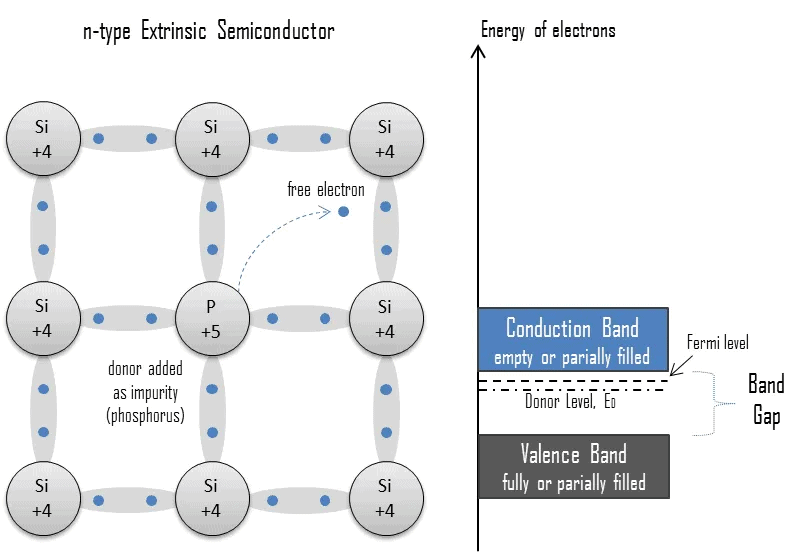• Mainly due to electrons
• Entirely neutral
• I = Ih and nh >> ne
• Majority – Electrons and Minority – Holes
• When a pure semiconductor (Silicon or Germanium) is doped by pentavalent impurity (P, As, Sb, Bi) then, four electrons out of five valence electrons bonds with the four electrons of Ge or Si.
• The fifth electron of the dopant is set free. Thus, the impurity atom donates a free electron for conduction in the lattice and is called “Donar“.
• Since the number of free electron increases by the addition of an impurity, the negative charge carriers increase. Hence, it is called n-type semiconductor.
• Crystal as a whole is neutral, but the donor atom becomes an immobile positive ion. As conduction is due to a large number of free electrons, the electrons in the n-type semiconductor are the majority carriers and holes are the majority carriers.

P-Type Semiconductor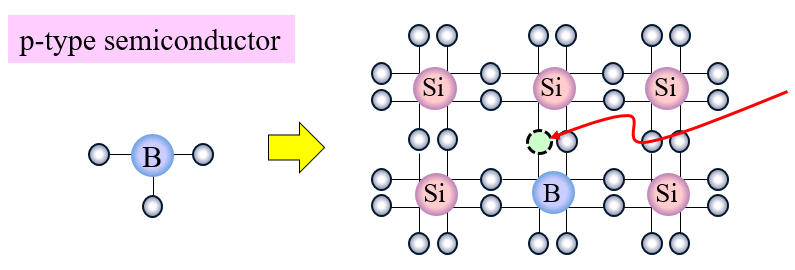• Mainly due to holes
• Entirely neutral
• I = Ih and nh >> ne
• Majority – Holes and Minority – Electrons
• When a pure semiconductor is doped with a trivalent impurity (B, Al, In, Ga ) then, the three valence electrons of the impurity bonds with three of the four valence electrons of the semiconductor.
• This leaves an absence of electron (hole) in the impurity. These impurity atoms which are ready to accept bonded electrons are called “Acceptors“.
• With the increase in the number of impurities, holes (the positive charge carriers) are increased. Hence, it is called p-type semiconductor.
• Crystal as a whole is neutral, but the acceptors become an immobile negative ion. As conduction is due to a large number of holes, the holes in the p-type semiconductor are majority carriers and electrons are majority carriers.

Table: Difference between N-type and P-type semiconductors

 S.No. N-type semiconductors P-type semiconductors 1. In these the impurity of some pentavalent element like P, As, Sb, Bi, etc. is mixed In these, the impurity of some trivalent element like b, Al, In, Ga etc. is mixed 2.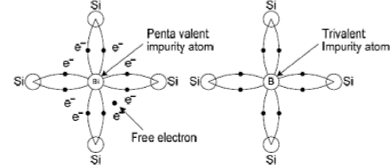3. in these the impurity atom donates one electrons, hence these are known as donor type semiconductors In these, the impurity atom can accept one electron, hence these are known as acceptor type semiconductors. 4. In these the electrons are majority current carriers and holes are minority current carriers, (i.e. the electron density is more than hole density nn >> np) In these the holes are majority current carriers and electrons are minority current carriers i.e. np >> nn 5. In these there is majority of negative particles (electrons) and hence are known as N-type semiconductors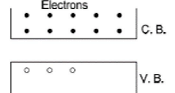In these there is majority of positive particles (cotters) and hence are known as P-type semiconductors.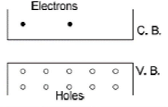6. in these the donor energy level is close to the conduction band and far away from valence band. In these the acceptor energy level is close to the valence band and far away from conduction band.

P N Junction Formation

(a) The device formed by joining atomically a wafer of P-type semiconductor to the wafer of N-type semiconductor is known as P-N junction.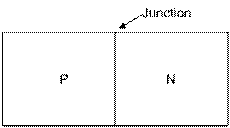(b)There are three processes of making junctions

(i) Diffusion

(ii) Alloying

(iii) Growth

In majority of cases P-N junction is formed by diffusion process. The impurity concentration is maximum at surface and decreases gradually inside the semiconductor.

(c) Conduction of current in P-N Junction: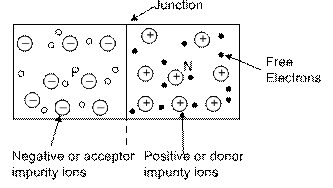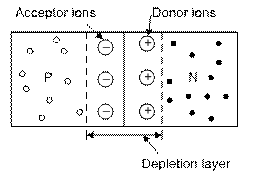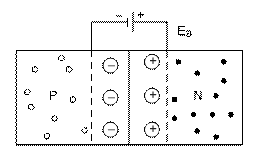(i) In P-N junction the majority cotters in P-region and majority electrons in N-region start diffusing due to concentration gradient and thermal disturbance towards N-region and P-region respectively and combine respectively with electrons and cotters and become neutral.

(ii) In this process of neutralization there occurs deficiency of free current carriers near the junction and layers of positive ions in N-region and negative ions in P-region are formed. These ions are immobile. Due to this an imaginary battery or internal electric field is formed at the junction which is directed from N to P.

(a) The region on both sides of P-N junction in which there is deficiency of free current carriers, is known as the depletion layer.

(b) Its thickness is of the order of 1 micro m (= 10–6)

(c) On two sides of it, there are ions of opposite nature. i.e. donor ion (+ve) on N-side and acceptor ions (–ve) on P-side.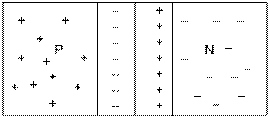(d) This stops the free current carriers to crossover the junction and consequently a potential barrier is formed at the junction.

(e) The potential difference between the ends of this layer is defined as the contact potential or potential barrier (VB).

(f) The value of VB is from 0.1 to 0.7 volt which depends on the temperature of the junction. It also depends on the nature of semiconductor and the doping concentration. For germanium and silicon its values are 0.3 V and 0.7 V respectively.

(h) Symbolic representation of diode: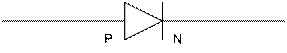(ii) The direction of current flow is represented by the arrow head.

(iii) In equilibrium state current does not flow in the junction diode.

(iv) In can be presumed to be equivalent to a condenser in which the depletion layer acts as a dielectric.(v) Potential distance curve at P-N Junction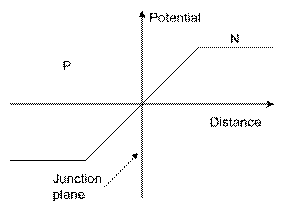(vi) Charge density curve at P-N Junction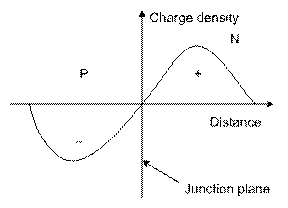(vii) Curve between electric field and distance near P-N junction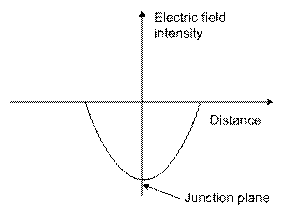The document Extrinsic Semiconductor: N-type & P-type Semiconductors - Notes | Study Physics Class 12 - NEET is a part of the NEET Course Physics Class 12.
All you need of NEET at this link: NEET

## Physics Class 12

157 videos|452 docs|213 tests
 Use Code STAYHOME200 and get INR 200 additional OFF

## Physics Class 12

157 videos|452 docs|213 tests

### How to Prepare for NEET

Read our guide to prepare for NEET which is created by Toppers & the best Teachers

Track your progress, build streaks, highlight & save important lessons and more!

,

,

,

,

,

,

,

,

,

,

,

,

,

,

,

,

,

,

,

,

,

;